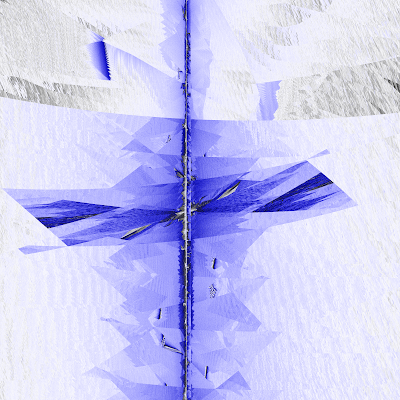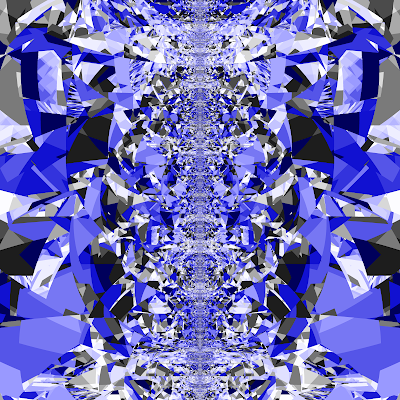Nelder-Mead method is a commonly used numeric method for solving optimization problems in several dimensions. Like many other iterative methods, it can produce interesting fractal images.
Like almost any numeric method for approximate solution, Nelder-Mead method requires the initial approximation to be specified. Speaking more precisely, for N-dimensional problem, this method requires N+1 initial point. Particularly, for 2-dimensional problems, 3 points must be specified.
In the cases, when the problem has several solutions, choice of the initial approximation determines (in some unpredictable way), which solution will be found, and some choices can give no convergence and no solution at all.
How initial choice relates to the convergence speed? The above picture shows that this relation is not an obvious one. Here is the recipe to obtain this picture:
• Consider the problem of minimizing the famous Rozenbrock banana function using Nelder-Mead algorithm.
• Use the following initial points: P1 = {0,-1}, P2={0,1}, and solve the problem for every point P3={x,y} withing the picture plane.
• Count, how many steps method would require to reach to the minimum {1,1} with some precision.
• Paint the pixels on the plane with different colors, according to the number of steps.
Here are more samples.
Minimizing the Rozenbrock function:Альбом: Math

Zoomed fragment of the above:Альбом: Math

Function with two minimums: f(x,y)=((x-1)2+y2)((x+1)2+y2)Альбом: Math

Simple function with 1 minimum at (0,0): f(x,y) = x2+y2Альбом: Math